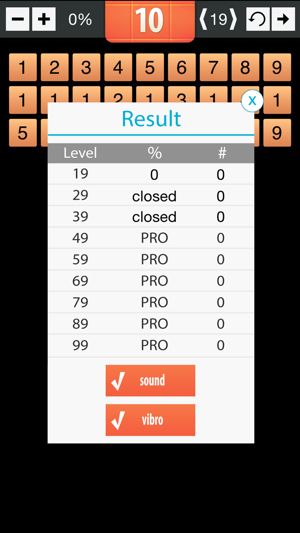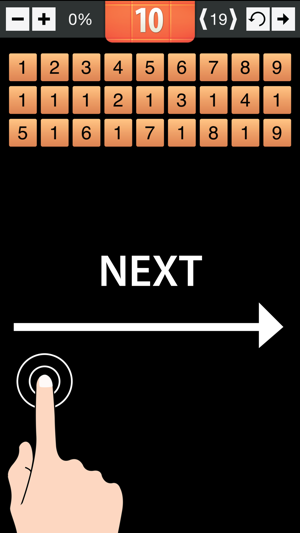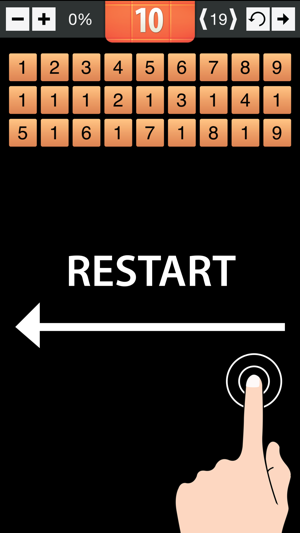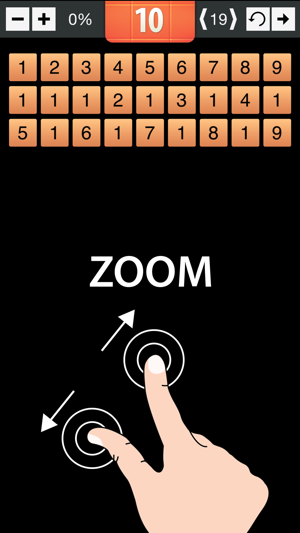## Screenshots

•••••## Description

Rules of the game: it is necessary to cross out all the numbers by two numbers equal to each other or equal to 10 (ten).

Example:
1) 1=1, 2=2, 3=3, 4=4, 5=5, 6=6, 7=7 ,8=8,9=9;
2) 1+9=10, 2+8=10, 3+7=10, 4+6=10;
3) 6+4=10, 7+3=10, 8+2=10, 9+1=10.

Web site: http://www.gm10.net

## What’s New

Version 1.2

Update for iPhone 6 and iPhone 6 plus

## Information

Provider
Vyacheslav Shishakin
Size
3.1 MB
Category
Games
Compatibility

Requires iOS 6.1 or later. Compatible with iPhone, iPad and iPod touch.

Languages

English

Age Rating
4+
Price
Free

## Supports

•